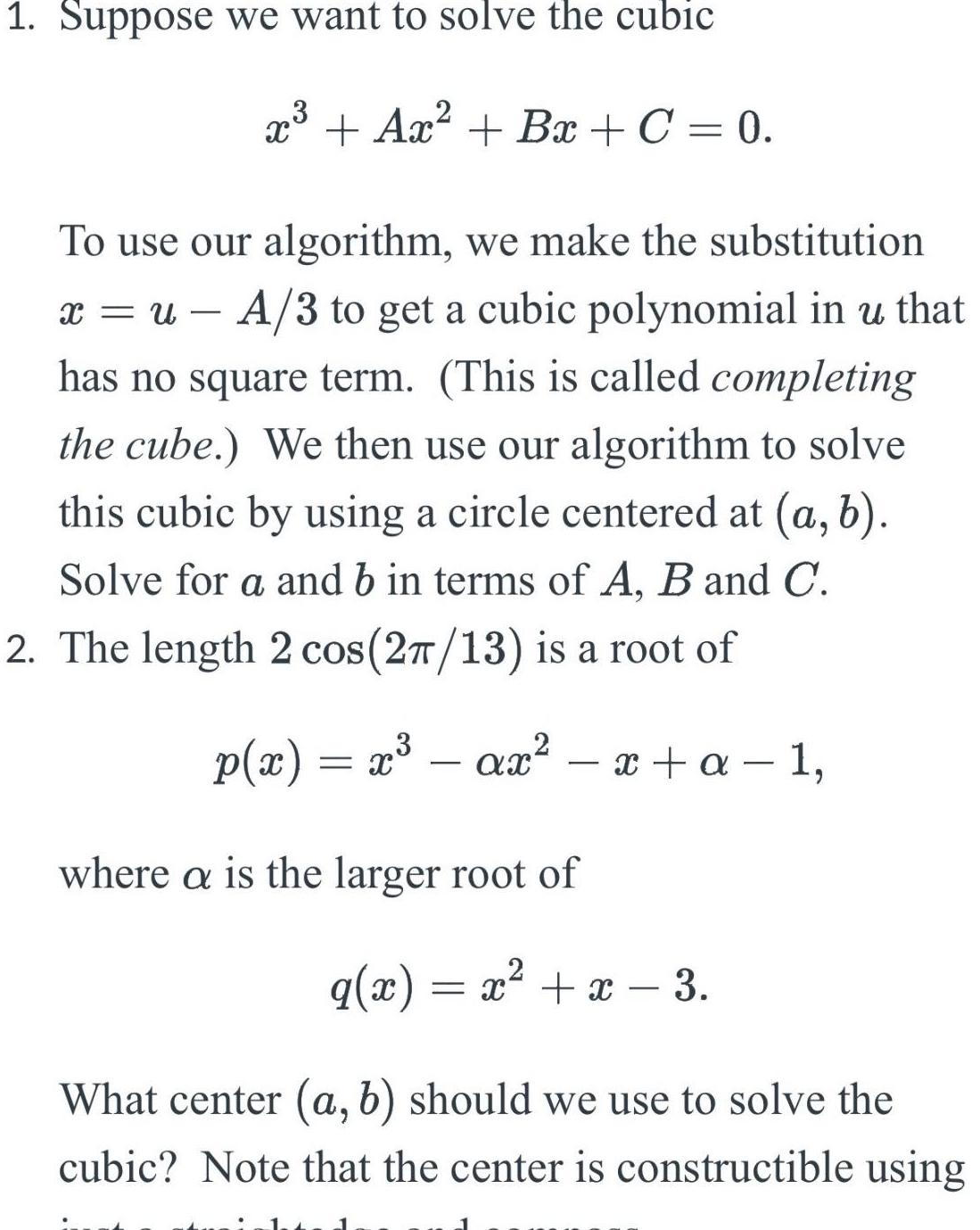Question:

# 1 Suppose we want to solve the cubic x Ax Bx C 0 To use our

Last updated: 7/26/20231 Suppose we want to solve the cubic x Ax Bx C 0 To use our algorithm we make the substitution x u A 3 to get a cubic polynomial in u that has no square term This is called completing the cube We then use our algorithm to solve this cubic by using a circle centered at a b Solve for a and b in terms of A B and C 2 The length 2 cos 2 13 is a root of p x x ax x a 1 where a is the larger root of q x x x 3 What center a b should we use to solve the cubic Note that the center is constructible using